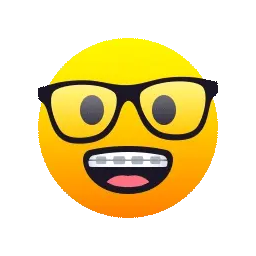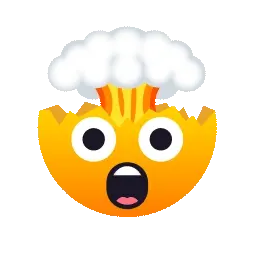Book Creator

# Pages 4 and 5 of 68

This is What We Have Learned 2Topic 8 and 12
Let’s learn!BY:JOSE CARLOS, SANTIAGO ORTIZ, LEANDRO MAURICIO AND JIMENA ZAPATA.
Topic 8
8 - 1 Basic Geometric Ideas
8 - 2 Measuring and Classifying Angles
8 - 3 Polygons
8 - 4 Triangles
8 - 6 Problem Solving: Make and Test Generalizations
8 - 1 Basic Geometric Ideas
Points and lines are basic geometric ideas. Lines are sometimes
described by relationships to other lines.If you see a line crossing another line it means they intersect.
In this example you can se line U intersects line P.
If you see this time a dot it means it is point (a letter or number).
In this example point J.Parallel lines can’t intersect because parallel means extending in the same direction, but without meeting.8-2
Measuring and Classifying AnglesRight
In geometry and trigonometry, a right angle is an angle of exactly 90 degrees or \pi /2 radians corresponding to a quarter turn. If a ray is placed so that its endpoint is on a line and the adjacent angles are equal, then they are right angles.
AcuteAn acute angle is an angle that is less than 90°, and these angles can be measured using a protractor.Obtuse
In Mathematics, “an obtuse angle is an angle which is greater than 90° and less than 180°”. In other words, an obtuse angle is between a right angle and a straight angle.Straight
A straight angle is a 180° angle.
8-3 polygons
A polygon is a closed plane figure made up of line
segments. Common polygons have names that tell
the number of sides the polygon has.Examples:OctagonHexagon
8-4 triangles
———————————————————————Examples:
equilateral triangleScalene triangleAcute triangleRight triangleParallelogram: a quadrilateral with both pairs of opposite sides parallel and equal in length.

Rectangle: a parallelogram with four right angles.

Rhombus: a parallelogram with all sides the same
length.

square: a rectangle with all sides the same length.

trapezoid: a quadrilateral with only one pair of parallel sides.————————————————————————————————————————————————————————————8-6 make and test generalizationsSay that triangles u,a,c and d,b,k are congruent?
Both triangles are congruent because each is a half of a square and squares have 4 congruent sides and angles.Topic 12
12 - 1 Using Customary Units of Length
12 - 2 Using Metric Units of Length
12 - 3 Perimeter
12 - 4 Area of Squares and Triangles
12 - 5 Area of Parallelograms
12 - 6 Area of Triangles
12 - 7 Circles and Circumference
12 - 8 Problem Solving: Draw a Picture and Make an Organized List
PrevNext